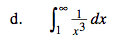### Home > CALC > Chapter Ch7 > Lesson 7.4.1 > Problem7-175

7-175.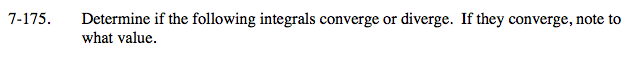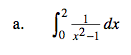Though you should recognize that this is an improper integral, finding the value to which it converges is a bit premature.
(You will develop this skill in Lesson 7.4.3.)
For that reason, the solution is provided for you.

By looking at the function, you should recognize that it has vertical asymptotes at both 1 and -1.
Therefore, you will have to evaluate the integral in two parts. But first we need to write the fraction as a sum of fractions with linear factors.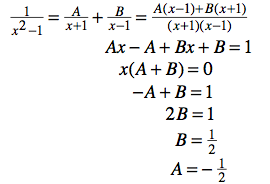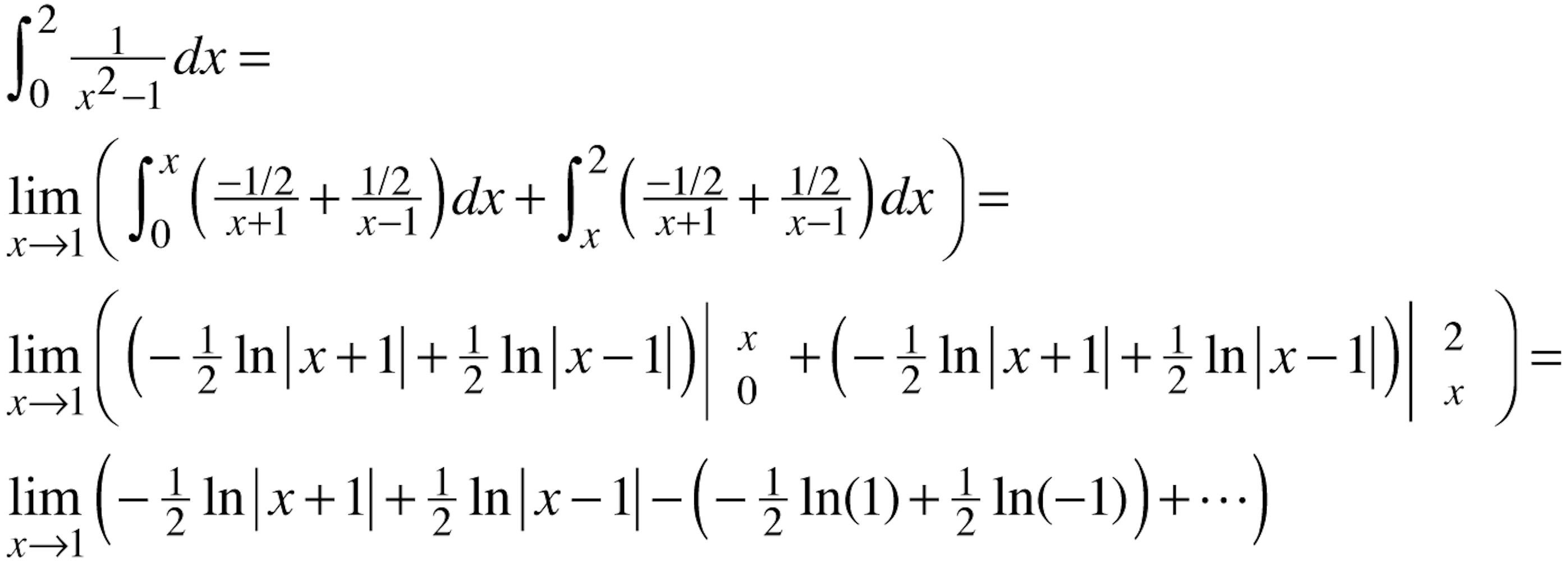Since ln(−1) does not exist, the integral diverges.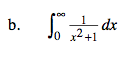Think inverse trig.
But also recognize that this is an improper integral.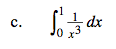Both integrands are the same in parts (c) and (d).
But one will converge and the other will diverge.
Analyze them carefully.